## Selina Concise Mathematics Class 6 ICSE Solutions Chapter 15 Decimal Fractions

Selina Publishers Concise Mathematics Class 6 ICSE Solutions Chapter 15 Decimal Fractions

APlusTopper.com provides step by step solutions for Selina Concise ICSE Solutions for Class 6 Mathematics. You can download the Selina Concise Mathematics ICSE Solutions for Class 6 with Free PDF download option. Selina Publishers Concise Mathematics for Class 6 ICSE Solutions all questions are solved and explained by expert mathematic teachers as per ICSE board guidelines.

IMPORTANT POINTS
Decimal Fraction : A fraction, whose denominator is 10 or a higher power of 10 e.g. 100, 1,000, 10,000 etc. is known as decimal fraction.
Number of Decimal Places: The number of digits in the decimal part of a number is the number of decimal places in it.
When the given number has only decimal part in it. It is always written 0 before it as 0.7, 0.55 are written as 0.7, 0.55.

Conversion of a Fraction into a Decimal Fraction :

1. When the denominator is 10,100,1000, 10,000 etc. : Counting from right to left of the numerator of the given fraction, mark the decimal point after as many digits as the number of zeroes in it denominator1. When the denominator is not, 10, 100, 1000, 10,000 etc.
Multiply both, the numerator and denominator of the given fraction, by a suitable number to get the denominator 10 or a power of 10 and then proceed as above, e.g.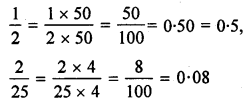2. Conversion of a given Decimal Fraction into a Non-Decimal Fraction : Remove the decimal point and at the same time write 1 in the denominator, as many zeroes to the right of 1 as there are digits in the decimal part e.g.,Zero or zeores written at the right of a decimal number does not change its value, e.g. 3.4 is the same as 3.40, 3.400, 3.4000 etc.

### Decimal Fractions Exercise 15A – Selina Concise Mathematics Class 6 ICSE Solutions

Question 1.
Write the number of decimal places in each of the following :
(i) 7.03
(ii) 0.509
(iii) 146.2
(iv) 0.0065
(v) 8.03207
Solution:
(i) 7.03, the decimal part is .03 which contains two digits.
Number 7.03 has 2 decimal places.
(ii) 0.509, the decimal part is 0.509 which contains three digits.
Number 0.509 has 3 decimal places
(iii) 146.2, the decimal part is .2 which contains one digits.
Number 146.2 has 1 decimal places.
(iv) 0.0065, the decimal part is .0065 which contains four digits.
Number 0.0065 has 4 decimal places
(v) 8.03207, the decimal part is .03207 which contains five digits.
Number 8.03207 has 5 decimal places.

Question 2.
Convert the given unlike decimal fractions into like decimal fractions:
(i) 1.36, 239.8 and 47.008
(ii) 507.0752, 8.52073 and 0.808
(iii) 459.22, 7.03093 and 0.200037
Solution:
(i) 1.36 = 1.360
239:8 = 239.800
47.008 = 47.008
(ii) 507.0752 = 507.07520
8.52073 = 8.52073
0.808 = 0.80800
(iii) 459.22 = 459.220000
7.03093 = 7.030930
0.200037 = 0.200037

Question 3.
Change each of following fractions to a decimal fraction :Solution: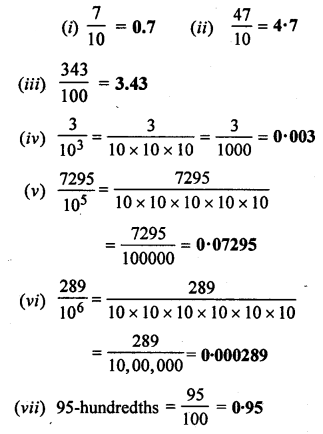Question 4.
Convert into a decimal fraction :Solution: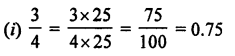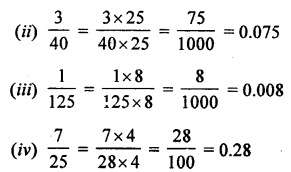Question 5.
Change the given decimals fractions to fractions in their lowest terms :
(i) 0.05
(ii) 3.95
(iii) 4.005
(iv) 0.876
(v) 50.06
(vi) 0.01075
(vii) 4.8806
Solution:### Decimal Fractions Exercise 15B – Selina Concise Mathematics Class 6 ICSE Solutions

Question 1.
(i) 0.243, 2.47 and 3.009
(ii) 0.0736, 0.6095 and 0.9107
(iii) 1.01, 257 and 0.200
(iv) 18, 200.35, 11.72 and 2.3
(v) 0.586, 0.0586 and 0.00586
Solution: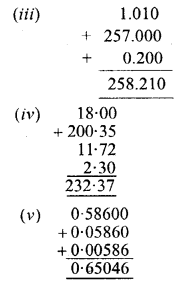Question 2.
Find the value of :
(i) 6.8 – 2.64
(ii) 2 – 1.0304
(iii) 0.1 – 0.08
(iv) 0.83 – 0.342
Solution: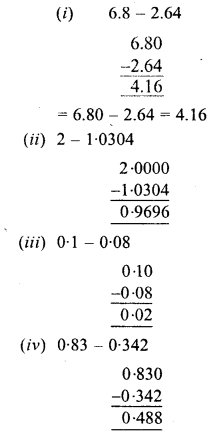Question 3.
Subtract :
(i) 0.43 from 0.97
(ii) 2.008 from 22.1058
(iii) 0.18 from 0.6
(iv) 1.002 from 17
(v) 83 from 92.05
Solution:
(i) 0.43 from 0.97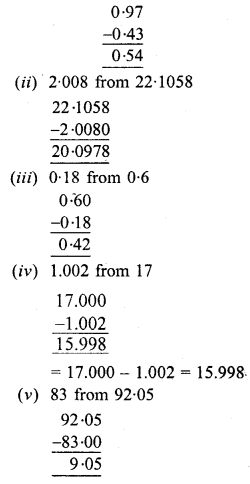Question 4.
Simplify :
(i) 3.5 – 2.43 + 0.075
(ii) 7.84 + 0.3 – 4.016
(iii) 2.987 – 1.25 – 0.54
(iv) 52.9 – 231.666 + 204
(v) 8.57 – 6.4432 – 1.70 + 0.683
Solution:
(i) 3.5 – 2.43 + 0.075
= 3.500 + 0.075 – 2.43
= 3.575 – 2.430 = 1.145
(ii) 7.84 + 0.3 – 4.016
= 7.840 + 0.300 – 4.016
= 8.140 – 4.016
= 4.124
(iii) 2.987 – 1.25 – 0.54
= 2.987 – 1.79
= 2.987 – 1.790
= 1.197
(iv) 52.9 – 231.666 + 204
= 52.9 – 231.666 + 204.0
= 256.9 – 231.666
= 256.900 – 231.666
= 25.234

Question 5.
From the sum of 75.75 and 4.9 subtract 28.465.
Solution: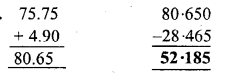Question 6.
Subtract the sum of 8.14 and 12.9 from 32.7.
Solution: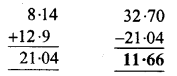Question 7.
Subtract the sum of 34.27 and 159.8 from the sum of 20.937 and 200.6.
Solution: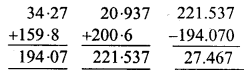Question 8.
From the sum of 2.43 and 4.349 subtract the sum of 0.8 and 3.15.
Solution:Question 9.
By how much does the sum of 18.0495 and 34.9644 exceed the sum of 7.6752 and 24.876 ?
Solution: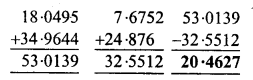Question 10.
What least number must be added to 89.376 to get 1000?
Solution: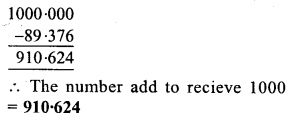### Decimal Fractions Exercise 15C – Selina Concise Mathematics Class 6 ICSE Solutions

Question 1.
Multiply :
(i) 5.6 and 8
(ii) 38.46 and 9
(iii) 0.943 and 62
(iv) 0.0453 and 35
(v) 7.5 and 2.5
(vi) 4.23 and 0.8
(vii) 83.54 and 0.07
(viii) 0.636 and 1.83
(ix) 6.4564 and 1000
(x) 0.076 and 100
Solution:
(i) 5.6 x 8 = 44.8
(ii) 38.46 x 9 = 346.14
(iii) 0.943 and 62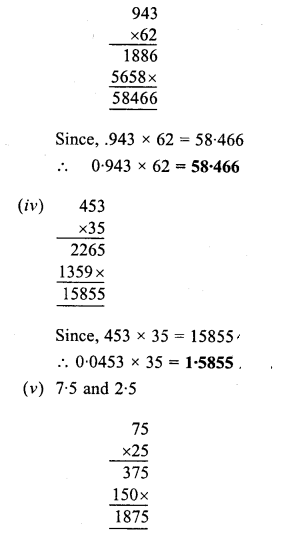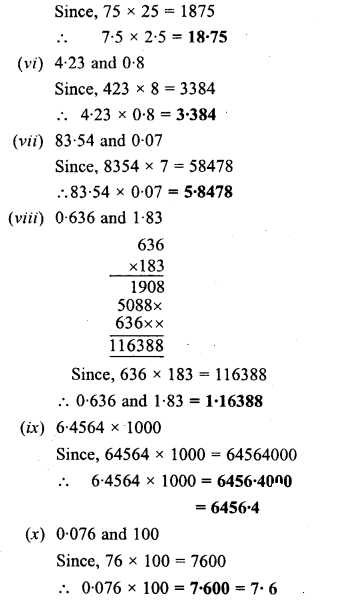Question 2.
Evaluate :
(i) 0.0008 x 26
(ii) 0.038 x 95
(iii) 1.2 x 2.4 x 3.6
(iv) 0.9 x 1.8 x 0.27
(v) 1.5 x 1.5 x 1.5
(vi) 0.025 x 0.025
(vii) 0.2 x 0.002 x 0.001
Solution:
(i) 0.0008 – 26
Since, 8 x 26 = 208
0.0008 x 26 = 0.0208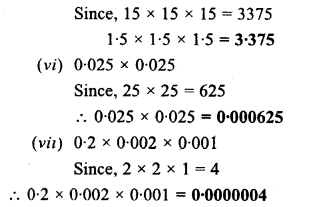Question 3.
Multiply each of the following numbers by 10, 100 and 1000 :
(i) 3.9
(ii) 2.89
(in) 0.0829
(iv) 40.3
(v) 0.3725
Solution:
(i) 3.9 x 10 = 39
3.9 x 100 = 390.0 = 390
3.9 x 1000 = 3900.0 =3900
(ii) 2.89 x 10 = 28.9
2.89 x 100 = 289
2.89 x 1000 = 2890.00 = 2890
(iii) 0.0829 x 10 = 0.829
0.0829 x 100 = 8.29
0.0829 x 1000 = 82.9
(iv) 40.3 x 10 = 403
40.3 x 100 = 4030
40.3 x 1000 = 40300
(v) 0.3725 x 10 = 3.725
0.3725 x 100 = 37.25
0.3725 x 1000 = 372.5

Question 4.
Evaluate :
(i) 8.64 ÷ 8
(ii) 0.0072 ÷ 6
(iii) 20.64 ÷ 16
(iv) 1.602 ÷ 15
(v) 13.08 ÷ 4
(vi) 3.204 ÷ 9
(vii) 3.024 ÷ 12
(viii) 5.15 ÷ 5
(ix) 3 ÷ 5
Solution:Question 5.
Divide each of the following numbers by 10,100 and 1000 :
(i) 49.79
(ii) 0.923
(iii) 0.0704
Solution: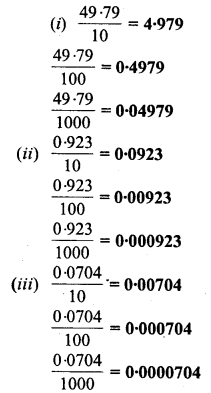Question 6.
Evaluate :
(i) 9.4 ÷ 0.47
(ii) 6.3 ÷ 0.09
(iii) 2.88 ÷ 1.2
(iv) 8.64 ÷ 1.6
(v) 37.188 ÷ 3.6
(vi) 16.5 ÷ 0.15
(vii) 3.2 ÷ 0.005
(viii) 3.24 ÷ 0.0016
Solution: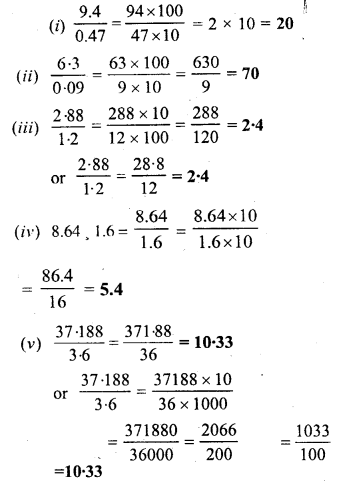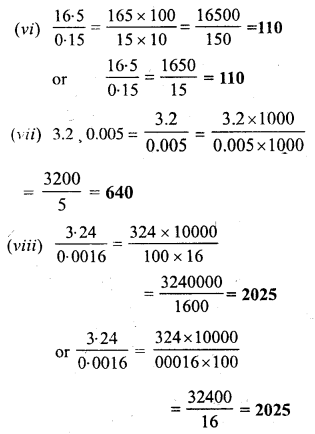Question 7.
Fill in the blanks with 10,100,1000, or 10000 etc.:
(i) 7.85 x ………….. = 78.5
(ii) 0.442 x ………. = 442
(in) 0.0924 x ………… = 9.24
(iv) 0.00187 x …………. = 18-7
(v) 2.6 x …………. = 2600
(vi) 0.08 x ………… = 80
(vii) 96.7 ÷ ………….. = 0.967
(viii) 5.2 ÷ ……………. = 0.52
(ix) 33.15 ÷ ……………….. = 0.03315
(x) 0.7 ÷ ………… = 0.007
(xi) 0.00672 x ……… = 67.2
Solution:
(i) 7.85 x 10 = 78.5
(ii) 0.442 x 1000 = 442
(iii) 0.0924 x 100 = 9.24
(iv) 0.00187 x 10000 = 18.7
(v) 2.6 x 1000 = 2600
(vi) 0.08 x 1000 = 80
(vii) 96.7 ÷ 100 = 0.967
(viii) 5.2 ÷ 10 = 0.52
(ix) 33.15 ÷ 1000 = 0.03315
(x) 0.7 ÷ 100 = 0.007
(xi) 0.00672 x 10000 = 67.2

Question 8.
Evaluate :
(i) 9.32 – 28.54 ÷ 10
(ii) 0.234 x 10 + 62.8
(iii) 3.06 x 100 – 889.4 ÷ 100
(iv) 2.86 x 7.5 + 45.4 ÷ 0.2
(v) 97.82 x 0.03 – 0.54 ÷ 0.3
Solution:
(i) 9.32 – 28.54 ÷ 10
= 9.32 – 2.854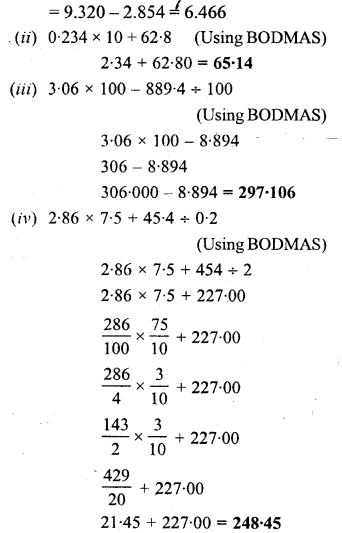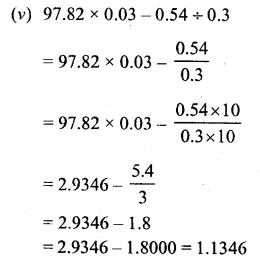### Decimal Fractions Exercise 15D – Selina Concise Mathematics Class 6 ICSE Solutions

Question 1.
Express in paise :
(i) Rs. 8.40
(ii) Rs. 0.97
(iii) Rs. 0.09
(iv) Rs. 62.35
Solution:
(i) Rs. 8.40 = 8.40 x 100 paise [1Rs. = 100 Paise]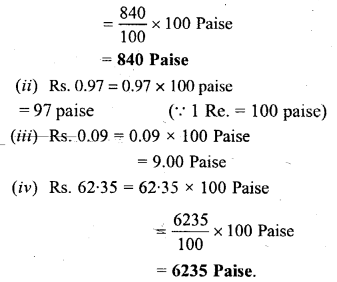Question 2.
Express in rupees :
(i) 55 P
(ii) 8 P
(iii) 695 P
(iv) 3279 P
Solution:Question 3.
Express in centimetre (cm) :
(i) 6 m
(ii) 8.54 m
(iii) 3.08 m
(iv) 0.87 m
(v) 0.03 m
(vi) 25.04 m
Solution:
(i) 6 x 100 = 600 cm
(ii) 8.54 x 100 = 854 cm
(iii) 3.08 x 100 = 308 cm
(iv) 0.87 x 100 = 87 cm
(v) 0.03 x 100 = 3 cm
(vi) 25.04 x 100 = 2504 cm

Question 4.
Express in metre (m) :
(i) 250 cm
(ii) 2328 cm
(iii) 86 cm
(iv) 4 cm
(v) 107 cm
Solution:Question 5.
Express in gramme (gm) :
(i) 6 kg
(ii) 5.543 kg
(iii) 0.078 kg
(iv) 3.62 kg
(v) 4.5 kg
Solution:
(i) 6 x 1000 = 6000 gm
(ii) 5.543 x 1000 = 5543 gm
(iii) 0. 078 kg = 0.078 x 1000 g = 78 g (1 kg = 1000 g)
(iv) 3.62 x 1000 = 3620 gm
(v) 4.5 x 1000 = 4500 gm

Question 6.
Express in kilogramme (kg) :
(i) 7000 gm
(ii) 6839 gm
(iii) 445 gm
(iv) 8 gm
(iv) 93 gm
(vi) 13545 gm
Solution: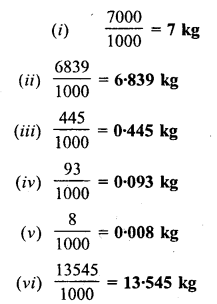Question 7.
(i) Rs. 5.37 and Rs. 12
(ii) Rs. 24.03 and 532 paise
(iii) 73 paise and Rs. 208
(iv) 8 paise and Rs. 1536
Solution: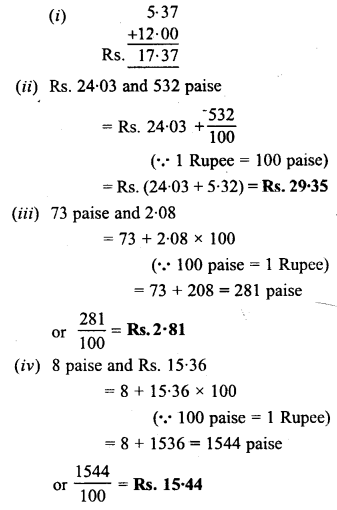Question 8.
Subtract :
(i) Rs. 35.74 from Rs. 63.22
(ii) 286 paise from Rs. 7.02
(iii) Rs. 0.55 from 121 paise
Solution: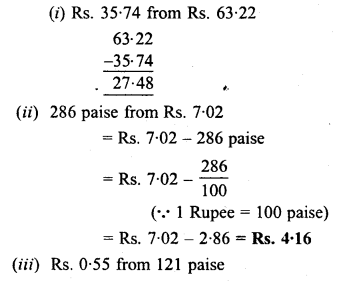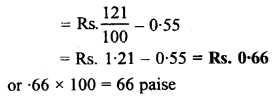Question 9.
(i) 2.4 m and 1.78 m
(ii) 848 cm and 2.9 m
(iii) 0.93 m and 64 cm
Solution:Question 10.
Subtract (giving answer in metre) :
(i) 5.03 m from 19.6 m
(ii) 428 cm from 1033 m
(iii) 0.84 m from 122 cm
Solution:Question 11.
(i) 2.06 kg and 57.864 kg
(ii) 778 gm and 1.939 kg
(iii) 0.065 kg and 4023 gm
Solution: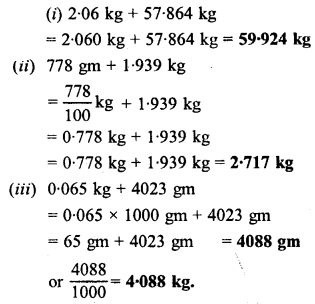Question 12.
Subtract (giving answer in kg) :
(i) 9.462 kg from 15.6 kg
(ii) 4317 gm from 23 kg
(iii) 0.798 kg from 4169 gm
Solution: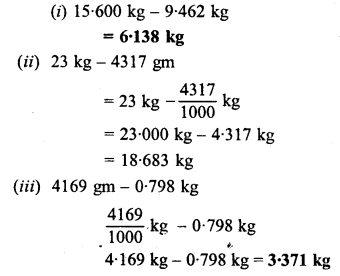### Decimal Fractions Exercise 15E – Selina Concise Mathematics Class 6 ICSE Solutions

Question 1.
The cost of a fountain pen is Rs. 13.25. Find the cost of 8 such pens.
Solution:
Cost of 1 fountain Pen = Rs. 13.25
Cost of 8 fountain Pen = 13.25 x 8 = 106.00 = Rs. 106

Question 2.
The cost of 25 identical articles is Rs. 218.25. Find the cost of one article.
Solution:Question 3.
The length of an iron rod is 10.32 m. The rod is divided into 4 pieces of equal lengths. Find the length of each piece.
Solution:
The length of iron rod = 10.32 m
Dividing in 4 equal parts = $$\frac { 10.32 }{ 4 }$$ = 2.58 m

Question 4.
What will be the total length of cloth required to make 5 shirts, if 2.15 m of cloth is needed for each shirt ?
Solution:
Cloth required for each shirt = 2.15 m
Cloth required for 5 shirts = 2.15 x 5 m = 10.75 m

Question 5.
Find the distance walked by a boy in 1$$\frac { 1 }{ 2 }$$ hours, if he walks at 2.150 km every hour.
Solution:Question 6.
83 note-books are sold at Rs. 15.25 each. Find the total money (in rupees) obtained by selling these note-books.
Solution:
Sale price of 1 note-book = Rs. 15.25
Sale of 83 books = Rs. 15.25 x 83 = Rs. 1265.75 paise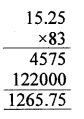Question 7.
If length of one bed-cover is 2.1 m, find the total length of 17 bed-covers.
Solution:
Length of one bed-cover = 2.1 m
Length of 17 bed-cover = 17 x 2.1 = 35.7 m

Question 8.
A piece of rope is 10 m 67 cm long. Another rope is 16 m 32 cm long. By how much is the second rope longer than the first one ?
Solution:
Length of one rope = 10 m 67 cm
Length of another rope = 16 m 32 cm
Difference in length = 16 m $$\frac { 32 }{ 100 }$$ cm – 10 m $$\frac { 67 }{ 100 }$$ cm
= 16.32 m – 10.67 m
= 5.65 m or 5 m 65 cm.

Question 9.
12 cakes of soap together weigh 5 kg and 604 gm. Find the weight of
(i) One cake in both kg and gramme
(ii) 5 cakes in kg.
Solution: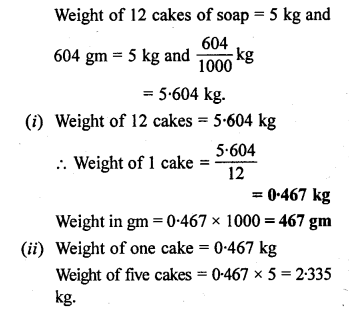Question 10.
Three strings of lengths 50 m 75 cm; 68 m 58 cm and 121 m 3 cm, respectively, are joined together to get a single string of greatest length, And the length of the single string obtained.
If this single string is then divided into 12 equal pieces ; find the length of each piece.
Solution:
1st string 50 m 75 cm = 50.75 m
2nd string 68 m 58 cm = 68.58 m
3rd string 121 m3 cm= 121.03 m
On joining three total length = 240.36 m
Now, one string = 240.36 m
Dividing 12 parts = $$\frac { 240.36 }{ 12 }$$ = 20.3 m.

### Decimal Fractions Revision Exercise – Selina Concise Mathematics Class 6 ICSE Solutions

Question 1.
Write th& following decimal numbers in ascending order of value
(i) 5.054, 5.250, 5.245 and 5.0543
(ii) 62.443, 62.434, 62.344 and 62.444
Solution:
(i) 5.054, 5.250, 5.245 and 5.0543
Writing them in like decimals :
5.0540, 5.2500, 5.2450, 5.0543
Now arranging in ascending order :
5.0540, 5.0543, 5.2450, 5.2500
=> 5.054 < 5.0543 < 5.245 < 5.250
(ii) 62.443, 62.434, 62.344 and 62.444
There are in like decimals :
Now writing in ascending order.
62.344, 62.434, 62.443, 62.444
or 62.344 < 62.434 < 62.443 < 62.444

Question 2.
What number added to 0.805 gives 1 ?
Solution:
The required number will be formed by subtracting 0.805 from 1
Required number = 1 – 0.805 = 1.000 – 0.805 = 0.195

Question 3.
What must be subtracted from 3 to get 2.462 ?
Solution:
The required number can be formed by subtracting 2.462 from 3
Required number = 3 – 2.462 = 3.000 – 2.462 = 0.538

Question 4.
By how much should 83.407 be decreased to get 27.78 ?
Solution:
The required number can be formed by subtracting 27.78 from 83.407
Required number = 83.407 – 27.78 = 83.407 – 27.780 = 55.627

Question 5.
Two articles weigh 32.674 kg and 40.038 kg respectively. Find :
(i) the total weight of both the articles.
(ii) the difference in the weights of both the articles.
Solution:
Weight of first article = 32.674 kg
Weight of second article = 40.038 kg
(i) Total weight of both the articles = (32.674 + 40.038) kg = 72.712 kg
(ii) Difference between the weights of the articles = (40.038 – 32.674) kg = 7.364 kg

Question 6.
By how much does the sum of 34.07 and 15.239 exceed the sum of 16.40 and 27.08?
Solution:
Sum of 34.07 and 15.239 = 34.070 + 15.239 = 49.309
and sum of 16.40 and 27.08 = 16.40 + 27.08 = 43.48
Difference between their sums = 49.309 – 43.48 = 49.309 – 43.480 = 5.829

Question 7.
The cost of 1 kg of fruit is Rs. 27.50. What is the cost of 3.6 kg of fruit ?
Solution:
Cost of 1 kg fruit = Rs. 27.50
Cost of 3.6 kg fruit = Rs. 27.50 x 3.6 = Rs. 99.00

Question 8.
Evaluate :
(i) 0.8 x 0.8 x 0.8
(ii) 0.8 ÷ 0.8 x 0.8
(iii) 0.8 x 0.8 ÷ 0.8
(iv) 0.8 ÷ 0.8 of 0.8
(v) 0.8 of 0.8 ÷ 0.8
Solution:
(i) 0.8 x 0.8 x 0.8 = 0.512
(ii) 0.8 ÷ 0.8 x 0.8Question 9.
Evaluate :
(i) 3.5 x (4.2 + 2.6)
(ii) 3.5 x 4.2 + 3.5 x 2.6
Are (i) and (ii) equal ?
Solution:
(i) 3.5 x (4.2 + 2.6) = 3.5 x (6.8) = 23.8
(ii) 3.5 x 4.2 + 3.5 x 2.6 = 14.7 + 9.1 = 23.8
Yes results of (i) and (ii) are equal.

Question 10.
Evaluate :
(i) (3.87 – 2.09) x 2.4
(ii) 3.87 x 2.4 – 2.09 x 2.4
Are (i) and (ii) equal ?
Solution:
(i) (3.87 – 2.09) x 2.4 = 1.78 x 2.4 = 4.272
(ii) 3.87 x 2.4 – 2.09 x 2.4 = 9.288 – 5.016 = 4.272
Yes, results of (i) and (ii) are equal.

Question 11.
A 4.85 m long pole is divided into 5 equal parts. Find the length of each part.
Solution:
Length of pole = 4.85 m
It is divided into 5 equal parts Length of each part = 4.85 ÷ 5 m = 0.97 m
Hence length of each part = 0.97 m

Question 12.
A car can run 16.8 km consuming one litre of petrol. How many kilometres will it run on 3.7 litres of petrol ?
Solution:
A car can go in one litre = 16.8 km
It will go in 3.7 litres of petrol = 16.8 x 3.7 km = 62.16 km

Question 13.
A certain amount of money is distributed among 28 persons. If each person gets Rs. 62.45 and Rs. 5.78 is left, find the original amount of money.
Solution:
Number of persons = 28
Each person gets = RS. 62.45
Total amount distributed to 28 persons = Rs. 62.45 x 28 = Rs. 1748.60
Amount left undistributed = Rs. 5.78
Total amount = Rs. 1748.60 + 5.78 = Rs. 1754.38

Question 14.
Complete the following table :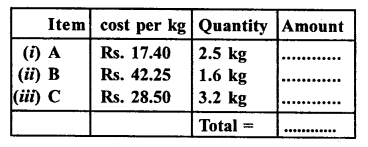Solution:
The given table has been completed as follows:Question 15.
The difference between two numbers is 47.364. If the smaller number is 31.855 ; find the bigger one.
Solution:
Difference of two number = 47.364
Smaller number = 31.855
Bigger number = 47.364 + 31.855 = 79.219